# 3. A fair coin is flipped eight times and the number of heads is counted. Calculate...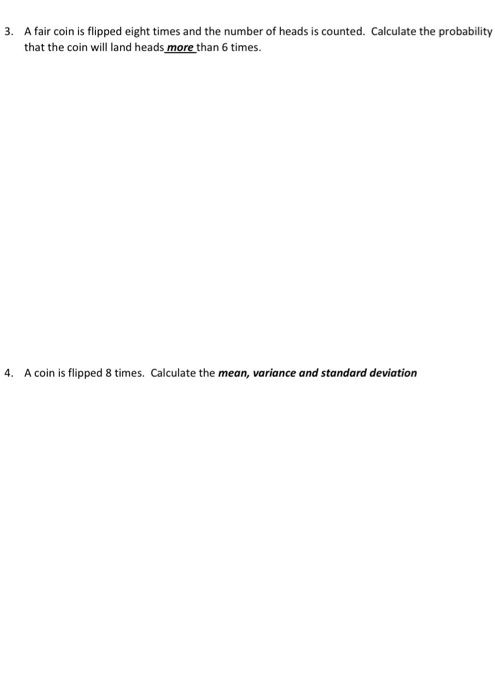3. A fair coin is flipped eight times and the number of heads is counted. Calculate the probability that the coin will land heads more than 6 times. 4. A coin is flipped 8 times. Calculate the mean, variance and standard deviation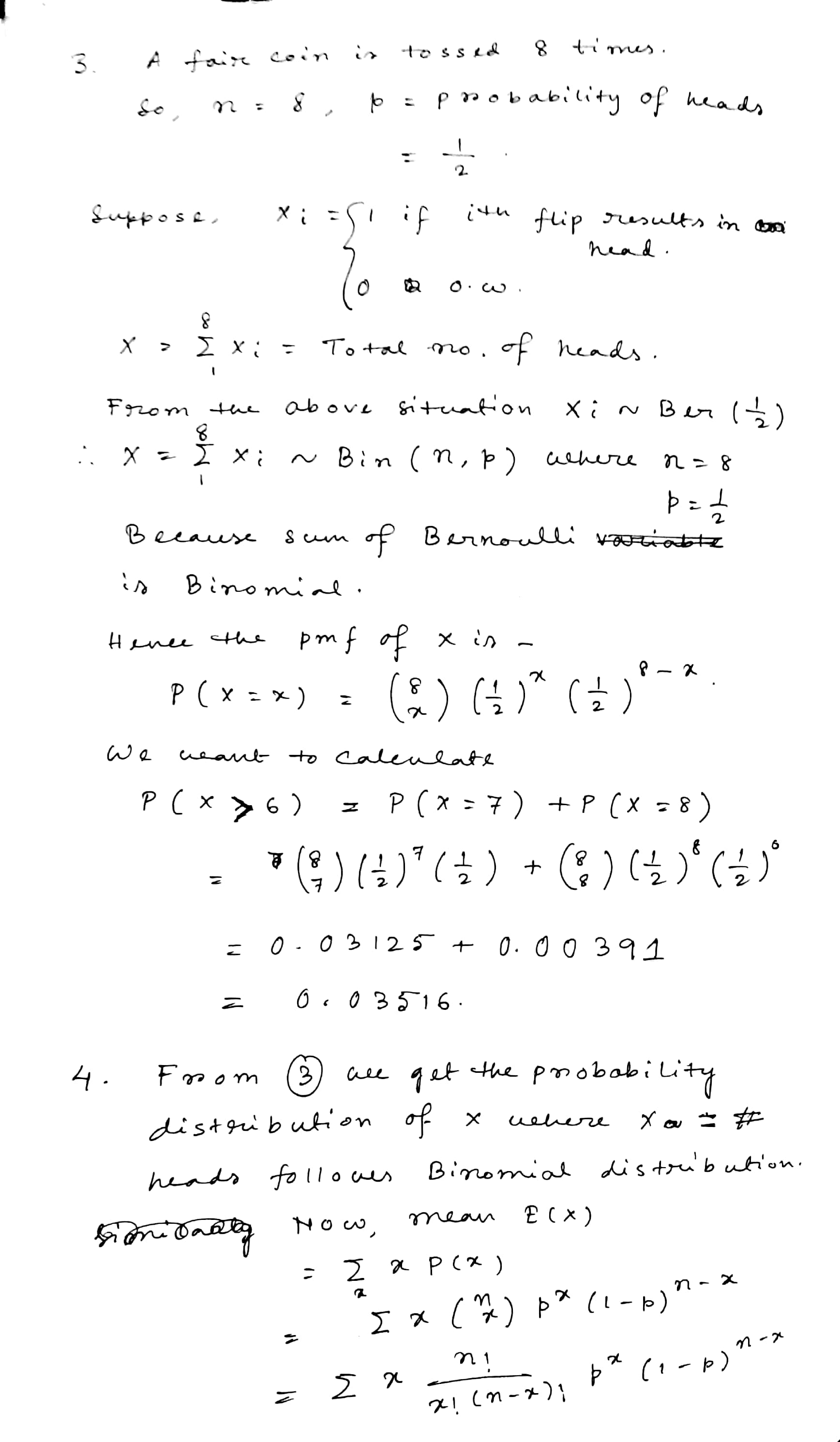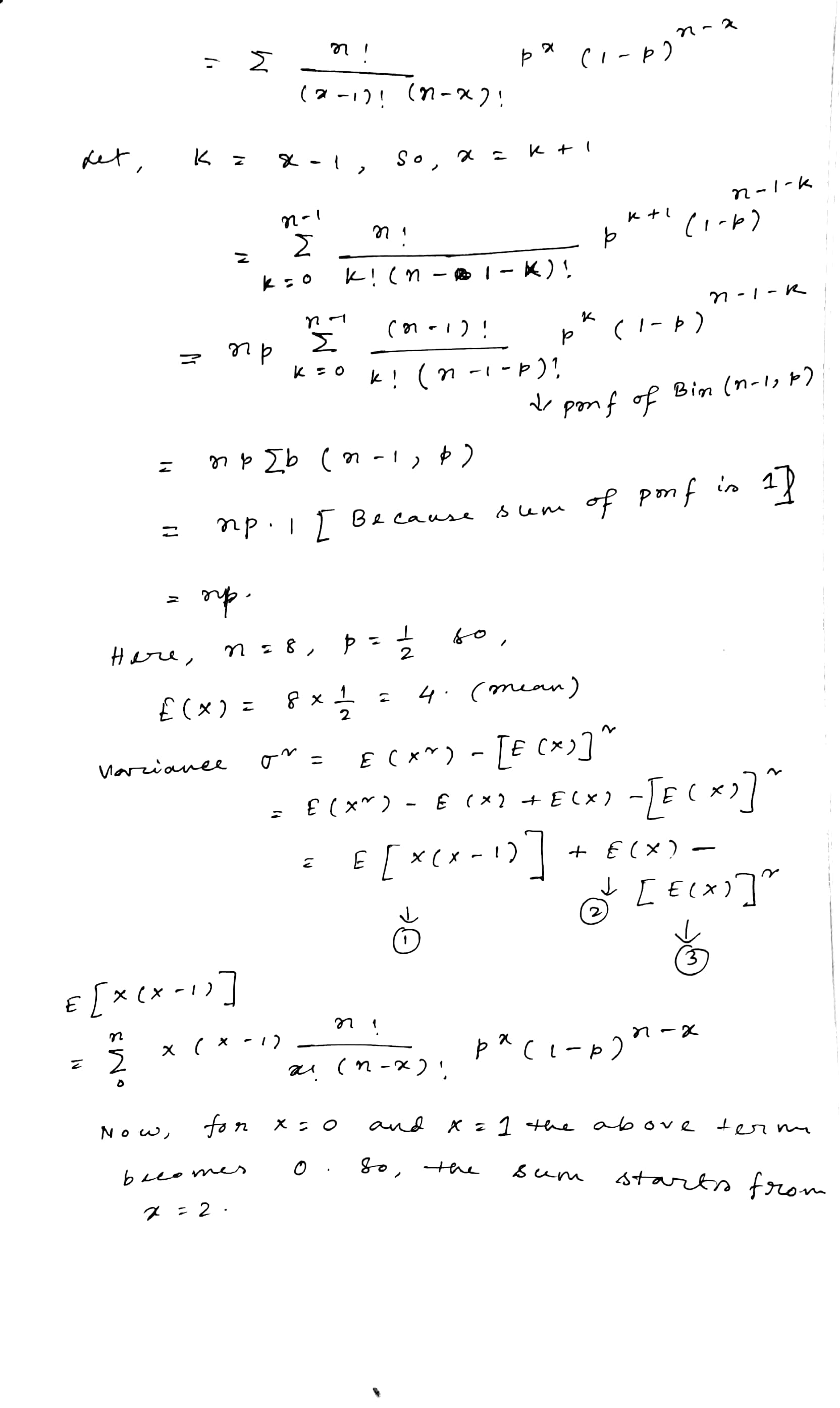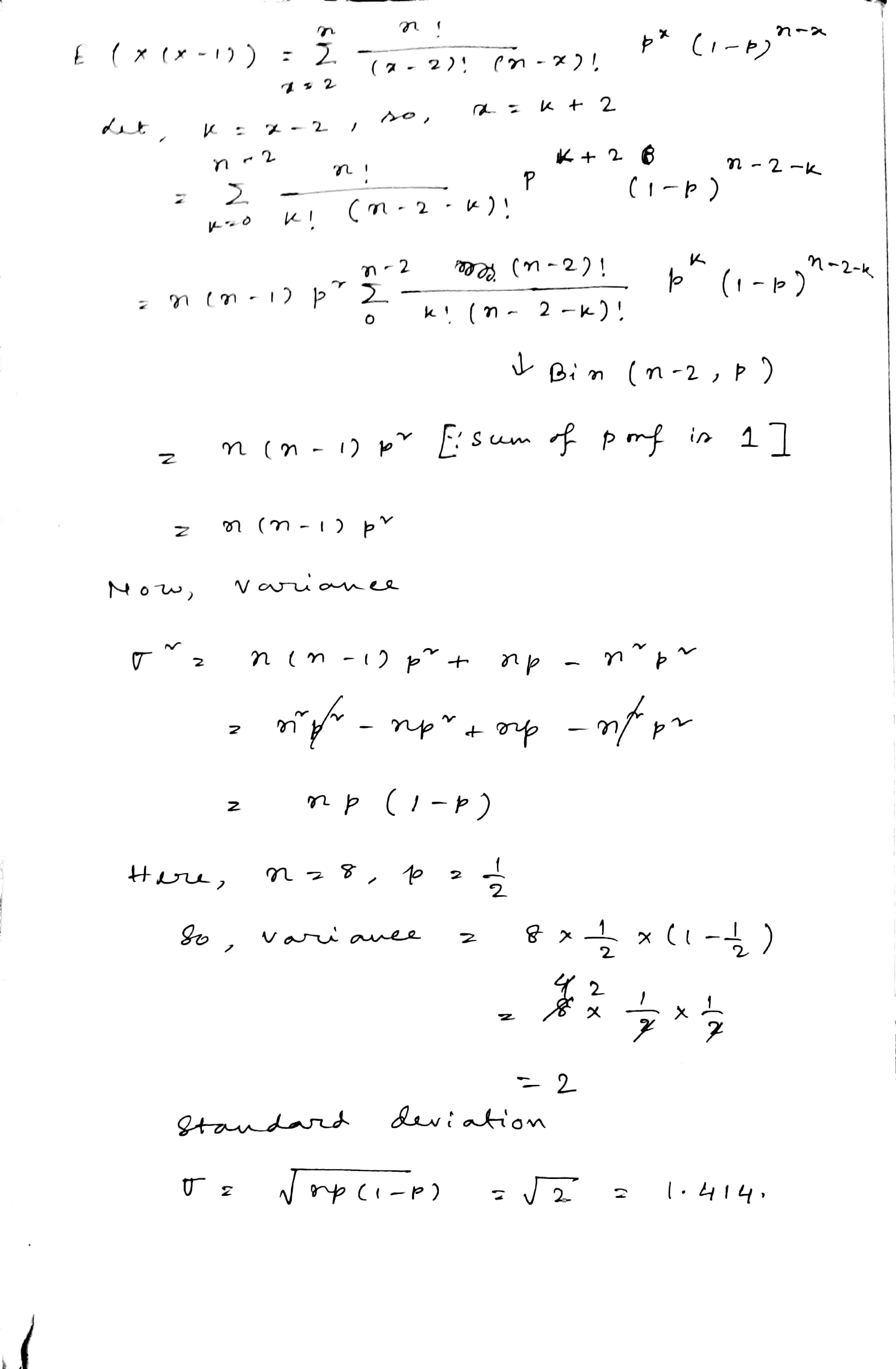##### Add Answer to: 3. A fair coin is flipped eight times and the number of heads is counted. Calculate...
Similar Homework Help Questions
• ### A fair coin is flipped 400 times. Calculate the mean and standard deviation for the number...

A fair coin is flipped 400 times. Calculate the mean and standard deviation for the number of heads. Calculate the mean and standard deviation for the proportion of heads. Calculate the approximate probability that the number of heads is at least 220.

• ### What is the probability of getting 2 heads when a fair coin is flipped 4 times?

What is the probability of getting 2 heads when a fair coin is flipped 4 times?

• ### 18. A fair coin is flipped multiple times until it lands on heads. If the probability...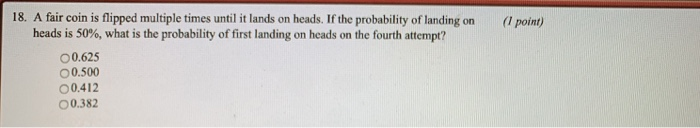18. A fair coin is flipped multiple times until it lands on heads. If the probability of landing on ( point) heads is 50%, what is the probability of first landing on heads on the fourth attempt? 00.625 0.500 00.412 00.382

• ### 7. A fair coin is flipped multiple times until it lands on heads. If the probability...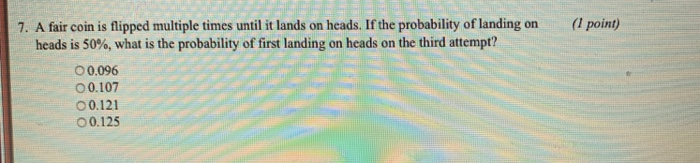7. A fair coin is flipped multiple times until it lands on heads. If the probability of landing on ( point) heads is 50%, what is the probability of first landing on heads on the third attempt? ○ 0,096 0.107 o 0.121 00.125

• ### 4. Toss a fair coin 6 times and let X denote the number of heads that...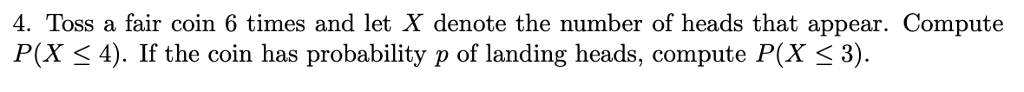4. Toss a fair coin 6 times and let X denote the number of heads that appear. Compute P(X ≤ 4). If the coin has probability p of landing heads, compute P(X ≤ 3) 4. Toss a fair coin 6 times and let X denote the number of heads that appear. Compute P(X 4). If the coin has probability p of landing heads, compute P(X < 3).

• ### A fair coin is tossed 10 times and the number of heads is counted. Complete parts​...

A fair coin is tossed 10 times and the number of heads is counted. Complete parts​ (a) through​ (d). a. Use the binomial distribution to find the probability of getting 5 heads. ​(Round to four decimal places as​ needed.) b. Use the binomial distribution to find the probability of getting at least 5 heads. ​(Round to four decimal places as​ needed.) c. Use the binomial distribution to find the probability of getting 5 to 7 heads. ​(Round to four decimal...

• ### You have flipped a fair coin 5 times and gotten a result of heads each time....

You have flipped a fair coin 5 times and gotten a result of heads each time. What can you tell me about the probability of getting a result of heads on the 6th flip?

• ### Answer part a and part b please!!! (a) What is the conditional probability that exactly four Tails appear w when a fair coin is flipped six times, given that the first flip came up Heads? (I.e. the co...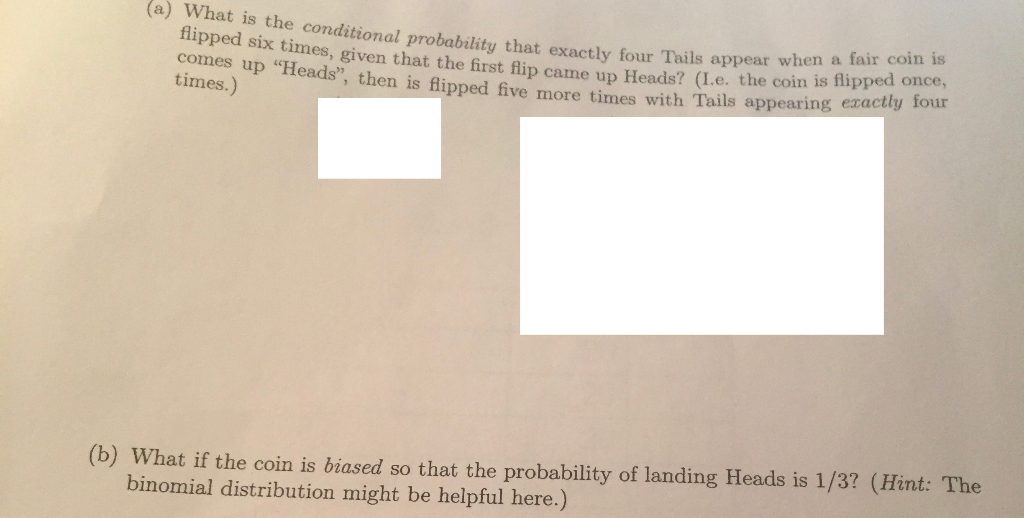Answer part a and part b please!!! (a) What is the conditional probability that exactly four Tails appear w when a fair coin is flipped six times, given that the first flip came up Heads? (I.e. the coin , then is flipped five more times with Tails appearing exactly lour times.) (b) What if the coin is biased so that the probability of landing Heads is 1/3? (Hint: The binomial distribution might be helpful here.) (a) What is the conditional...

• ### The probability of getting heads from throwing a fair coin is 1/2 The fair coin is...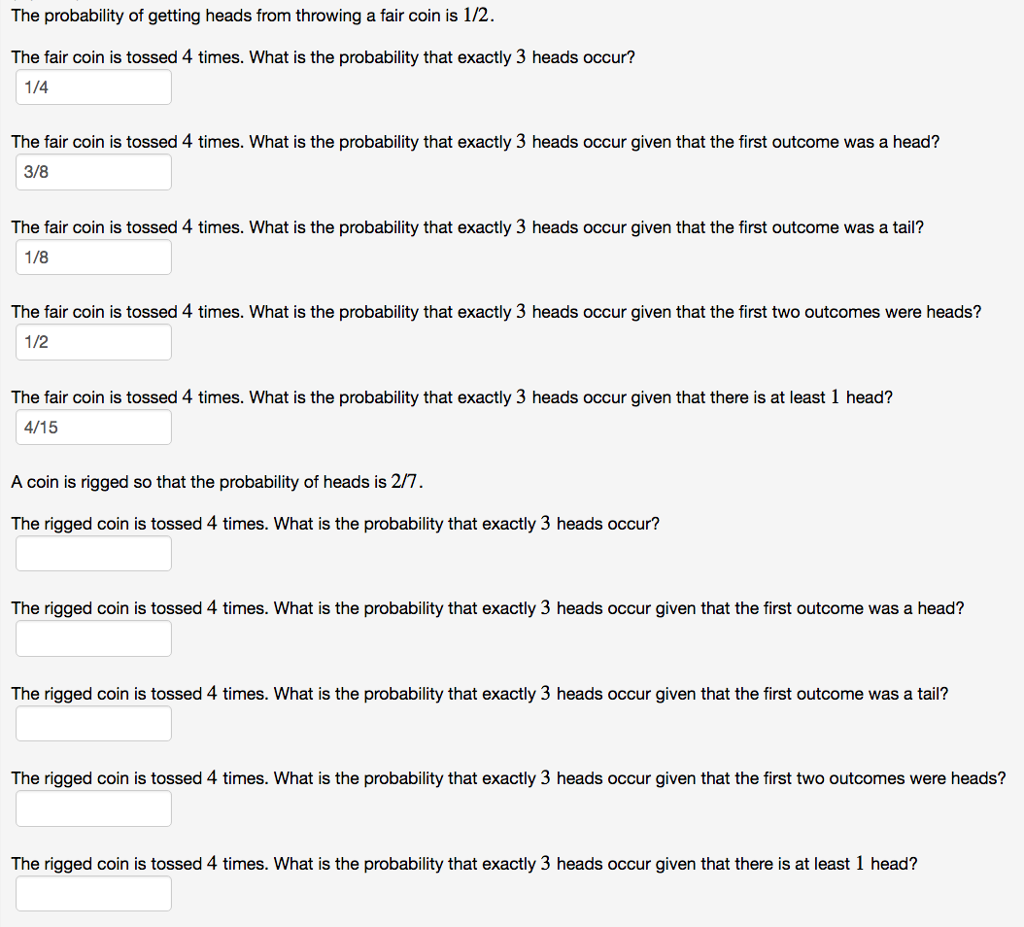The probability of getting heads from throwing a fair coin is 1/2 The fair coin is tossed 4 times. What is the probability that exactly 3 heads occur? 1/4 The fair coin is tossed 4 times. What is the probability that exactly 3 heads occur given that the first outcome was a head? 3/8 The fair coin is tossed 4 times. What is the probability that exactly 3 heads occur given that the first outcome was a tail? 1/8 The...

• ### 6. A fair coin is flipped repeatedly until 50 heads are observed. What is the probability...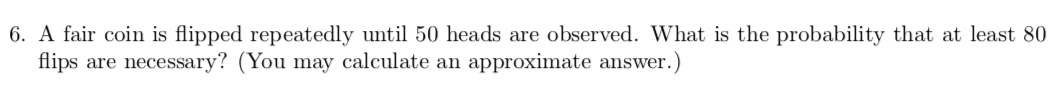6. A fair coin is flipped repeatedly until 50 heads are observed. What is the probability that at least 80 flips are necessary? (You may calculate an approximate answer.)

Free Homework App